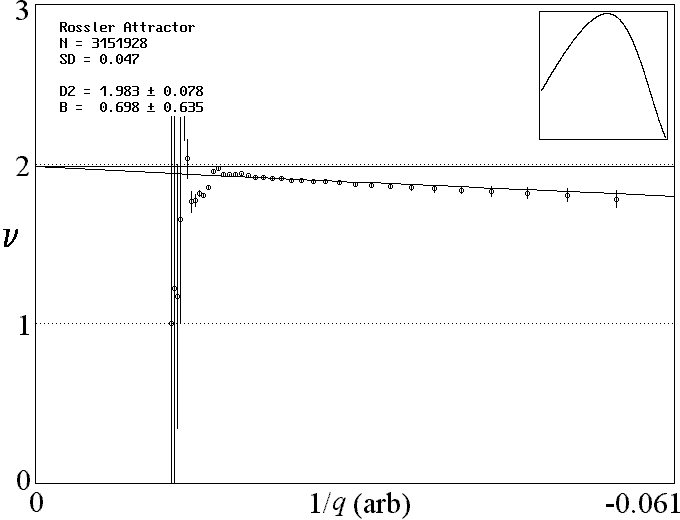# Improved Correlation Dimension Calculation

J. C. Sprott
Department of Physics, University of Wisconsin, Madison, WI 53706, USA

G. Rowlands
Department of Physics, University of Warwick, Coventry CV47AL, England

### ABSTRACT

For many chaotic systems, accurate calculation of the correlation dimension from measured data is difficult because of very slow convergence as the scale size is reduced. This problem is often caused by the highly nonuniform measure on the attractor. This paper proposes a method for collecting data at large scales and extrapolating to the limit of zero scale. The result is a vastly reduced required number of data points for a given accuracy in the measured dimension. The method is illustrated in detail for one-dimensional maps and then applied to more complicated maps and flows. Values are given for the correlation dimension of many standard chaotic systems.

Ref: J. C. Sprott, and G. Rowlands, International Journal of Bifurcation and Chaos 11, 1865-1880 (2001)

The complete paper is available in PDF format.

Fig. 1. Shapes of general symmetric maps for various alpha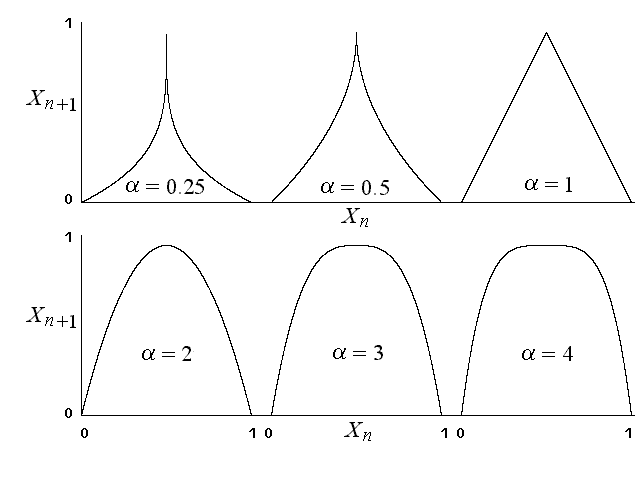Fig. 2. Probability density for the logistic map with A = 3.8.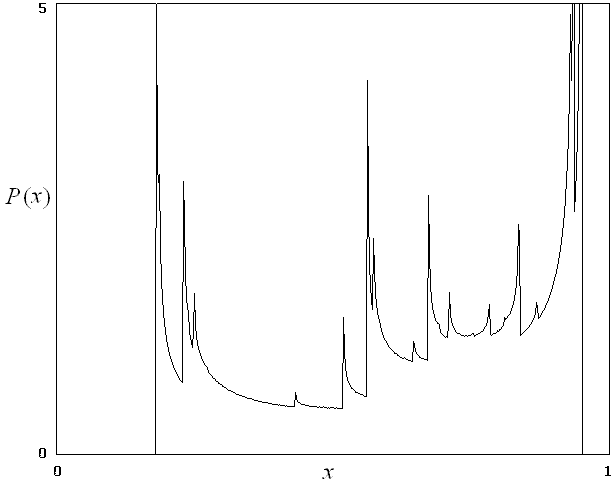Fig. 3. Average measure for the maximum x values for the Rossler attractor.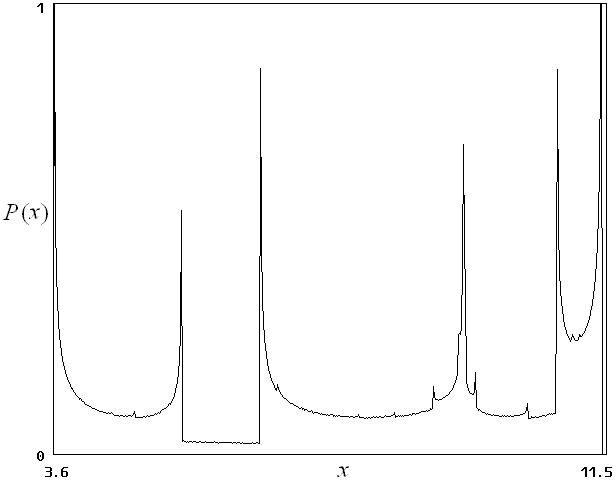Fig. 4. Plot showing slow convergence of the correlation dimension for the logistic map.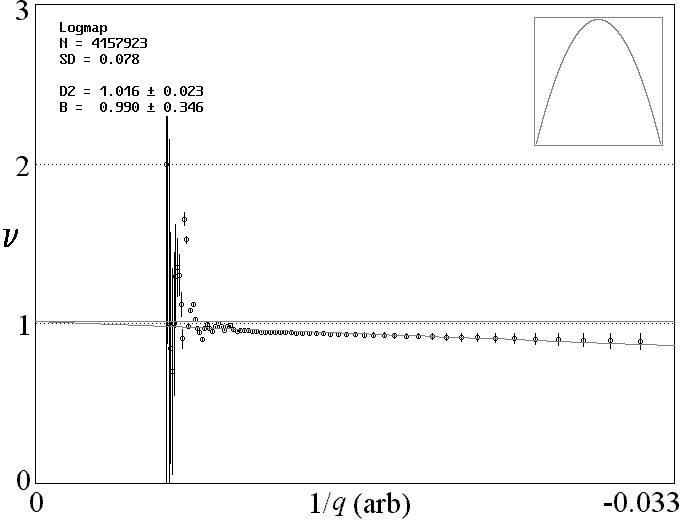Fig. 5. Calculated correlation dimension for the maps in Eq. (3) for various alpha.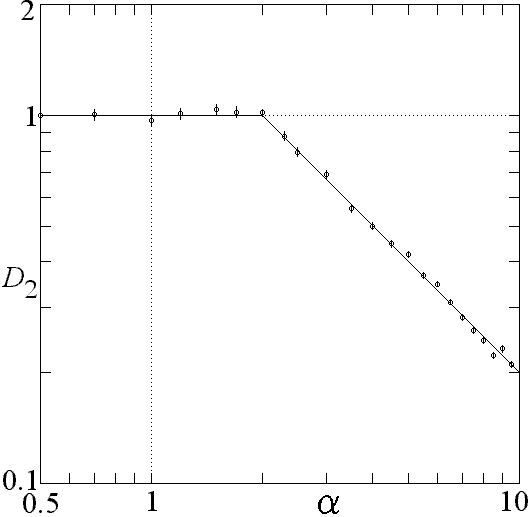Fig. 6. A measure of the slowness of convergence for the maps in Eq. (3) for various alpha.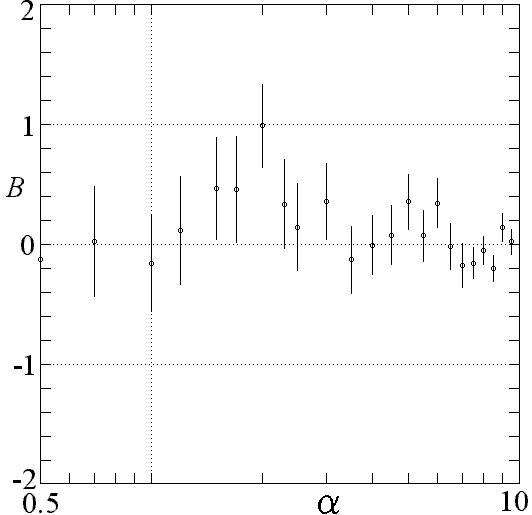Fig. 7. The Zaslavsky map switches back and forth from 1-D to 2-D depending on the scale.Fig. 8. Convergence of the correlation dimension for the Zaslavsky map shows large oscillations.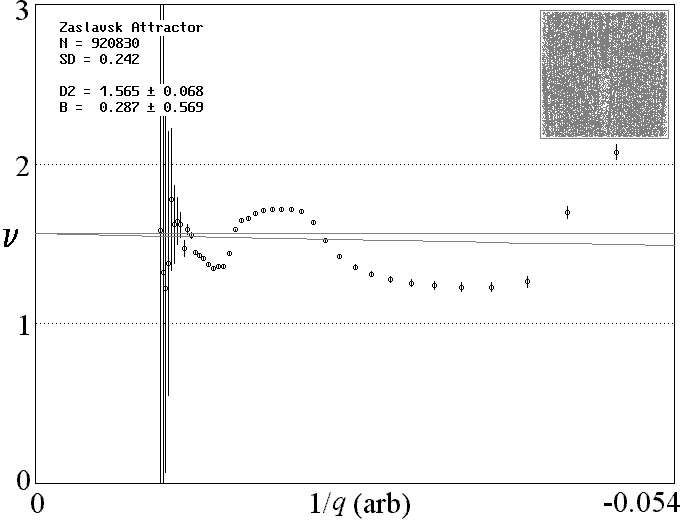Fig. 9. Convergence of the correlation dimension for the Rossler attractor resembles the logistic map.